# Selina Solutions Concise Maths Class 7 Chapter 12: Simple Linear Equations (Including Word Problems)

Selina Solutions Concise Maths Class 7 Chapter 12 Simple Linear Equations (Including Word Problems) contains answers in a comprehensive manner for a better understanding among students. The solutions help students verify their answers and get an idea about the steps to be followed in solving problems easily. Here, the students can find Selina Solutions Concise Maths Class 7 Chapter 12 Simple Linear Equations (Including Word Problems) free PDF, with the help of links provided for free download.

Chapter 12 explains the method of solving equations, which contain fractions, transposing terms and word problems. The problems are solved by subject matter experts who possess vast experience in the respective fields.

## Selina Solutions Concise Maths Class 7 Chapter 12: Simple Linear Equations (Including Word Problems) Download PDF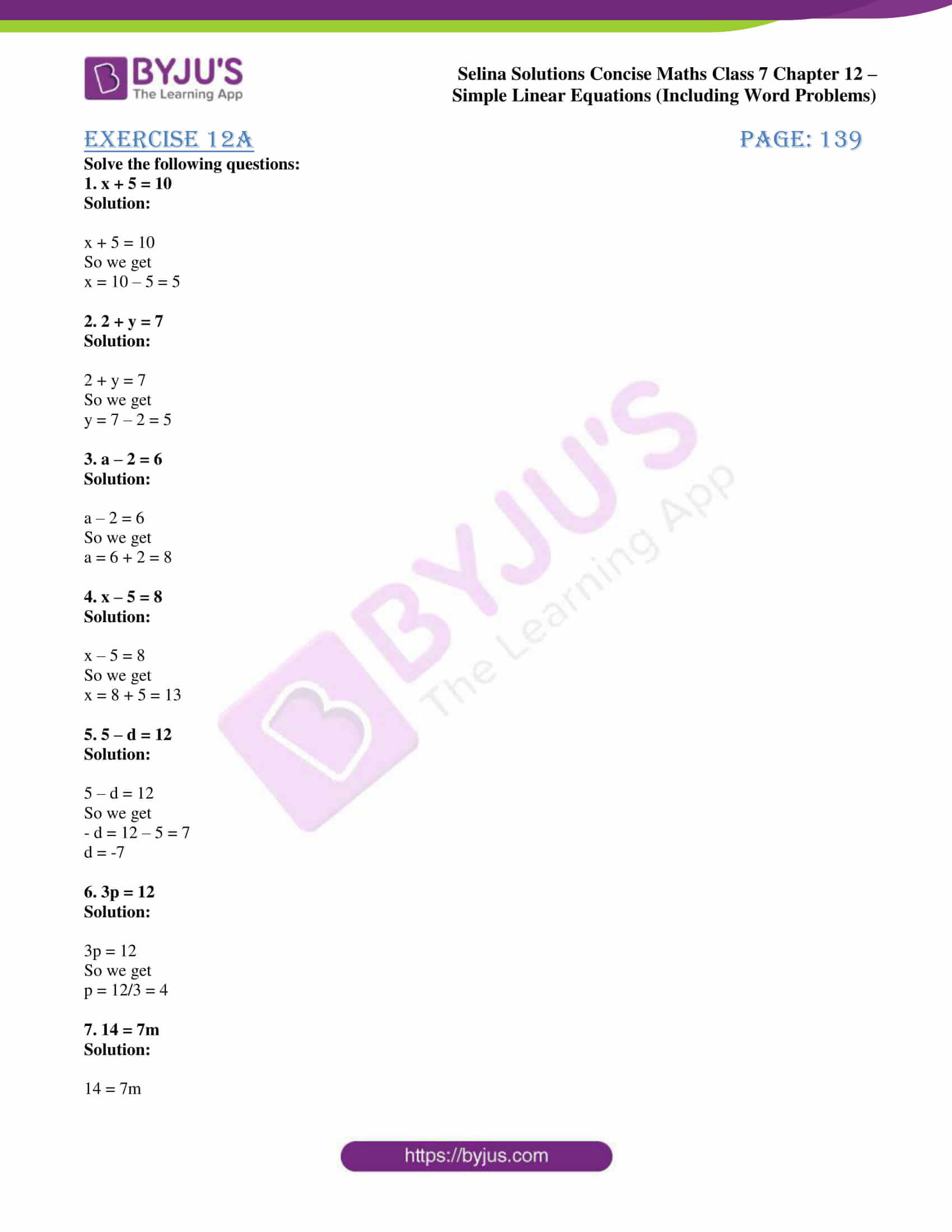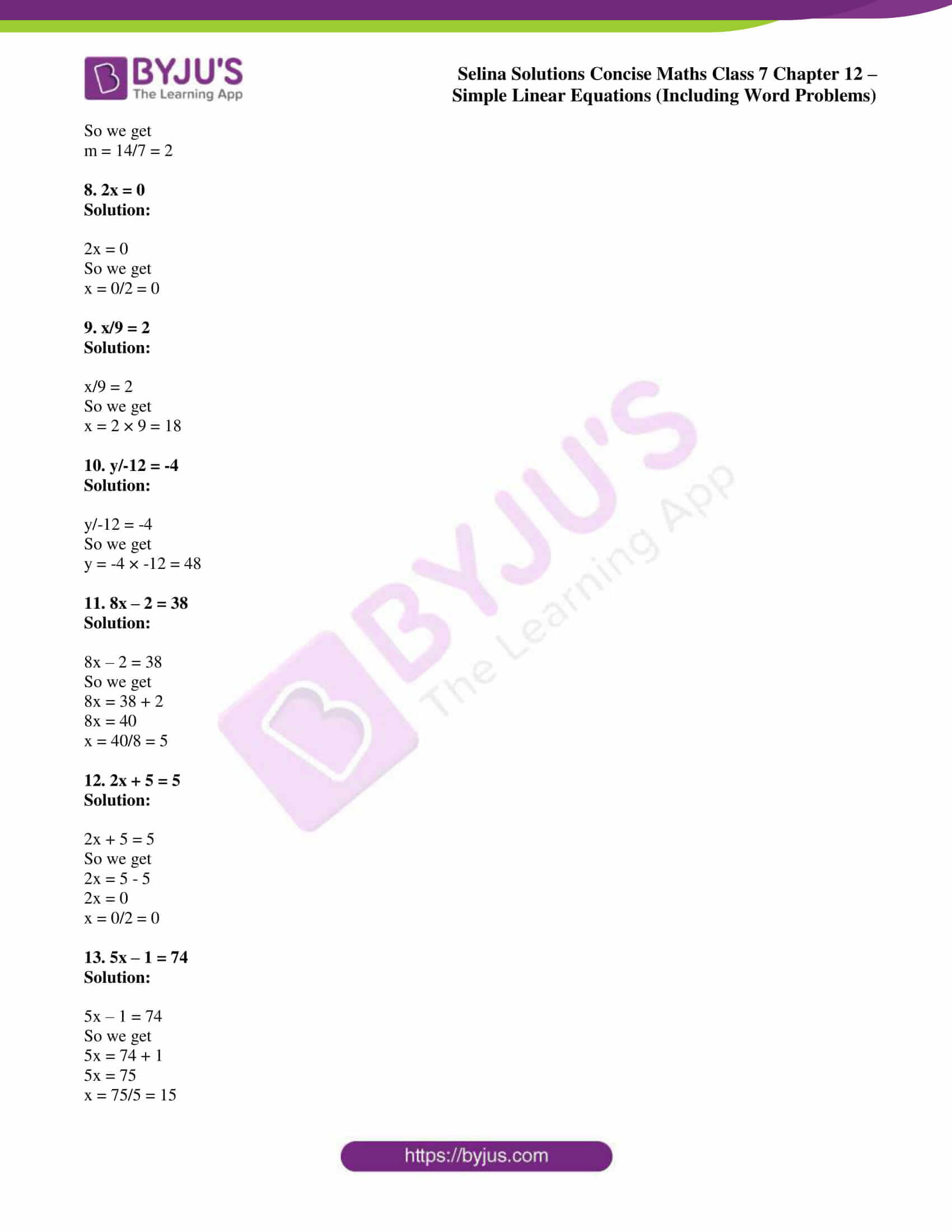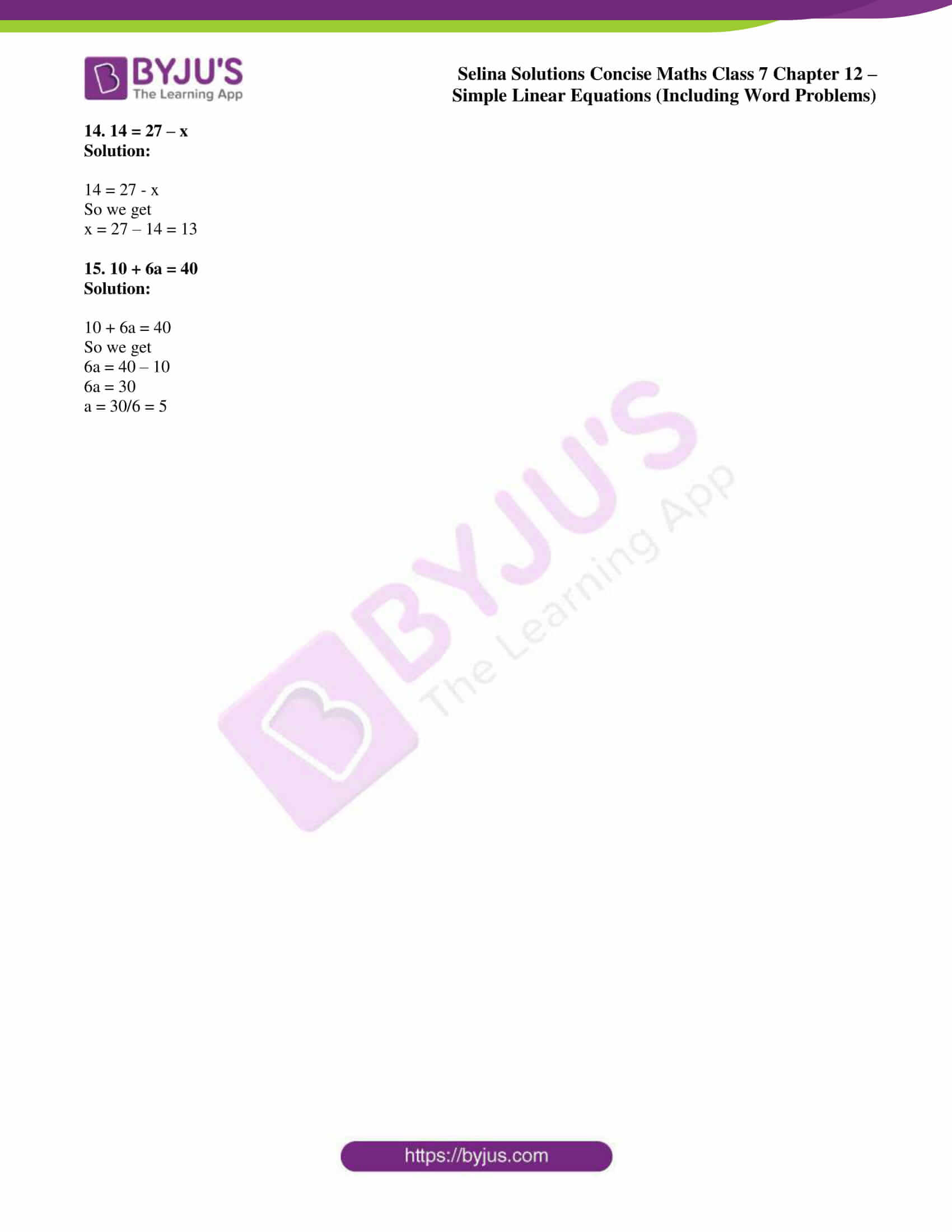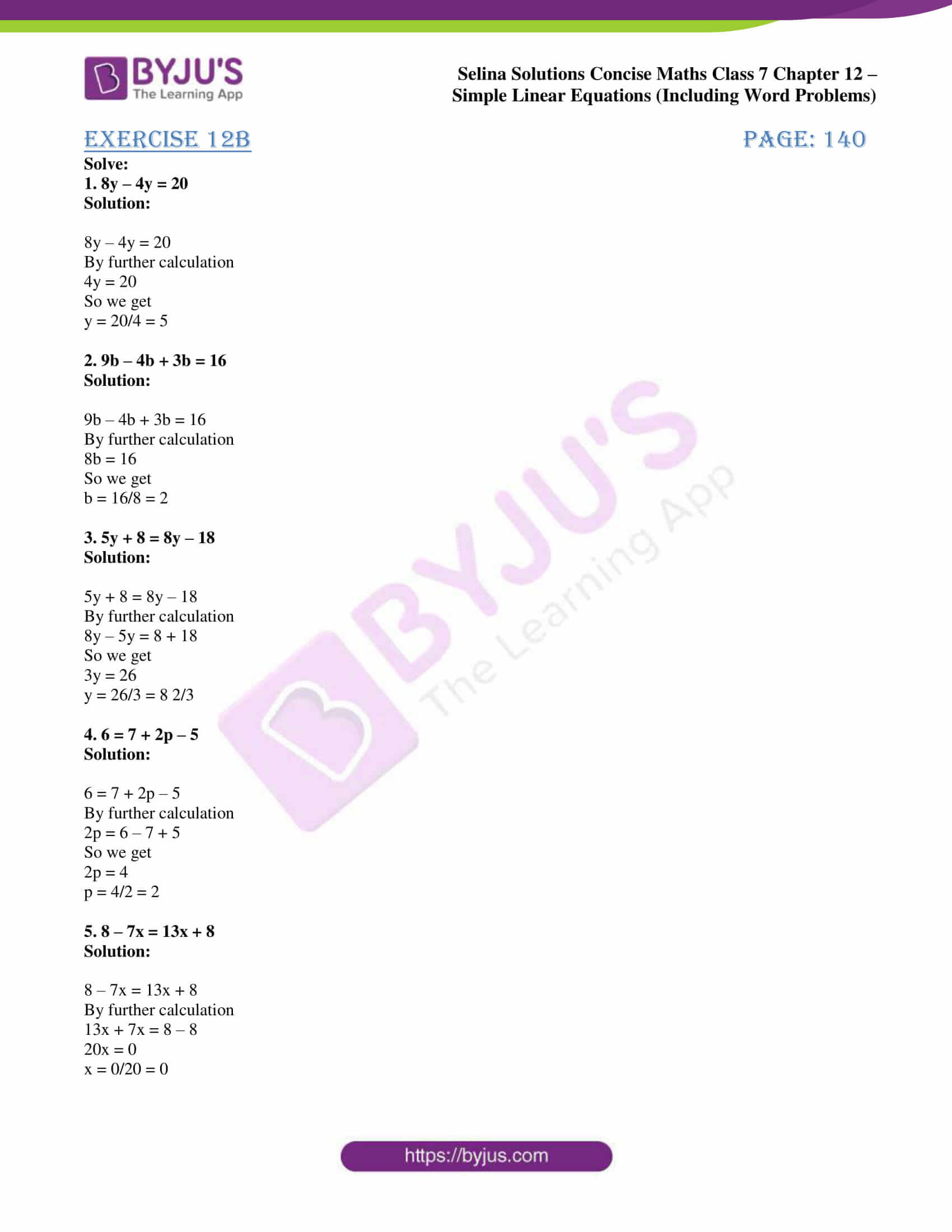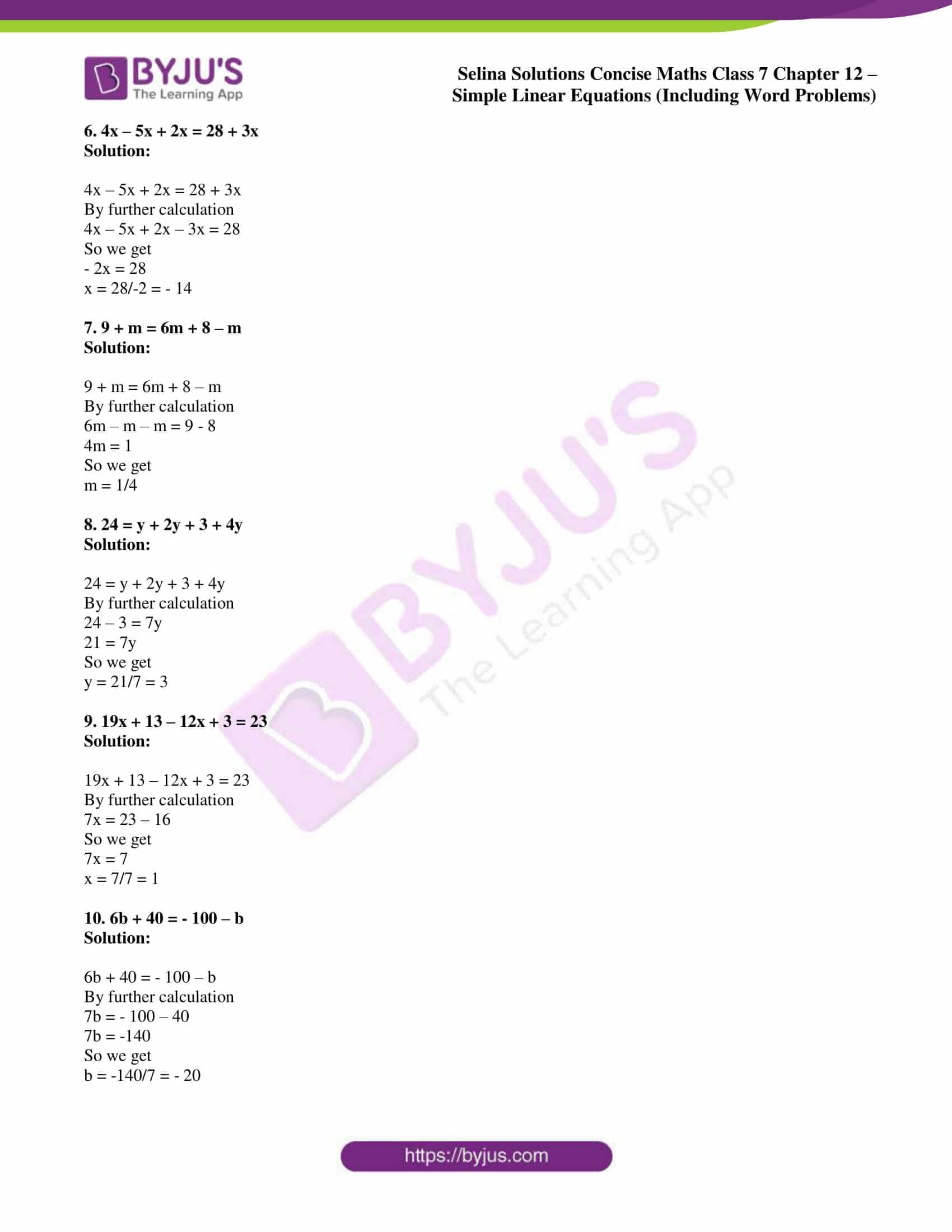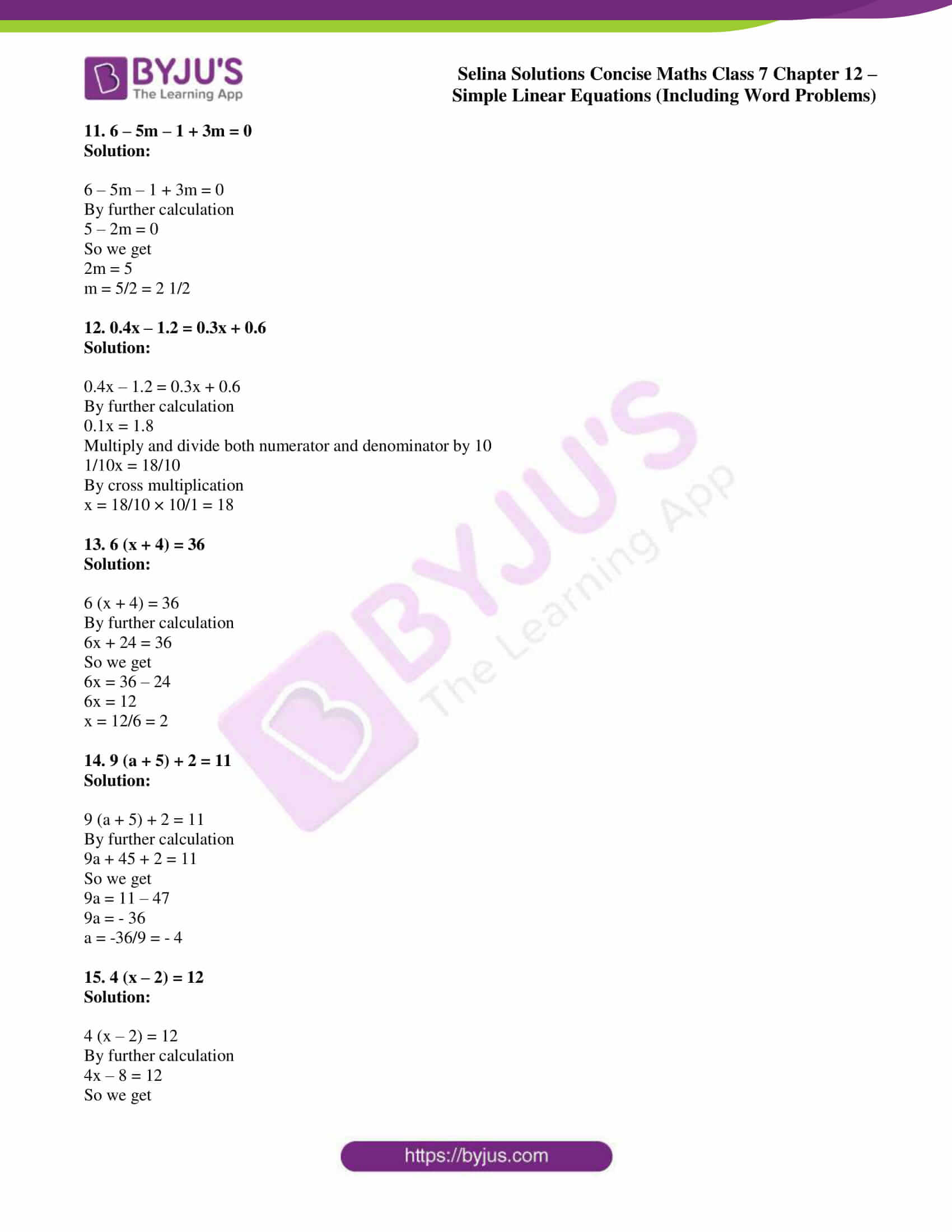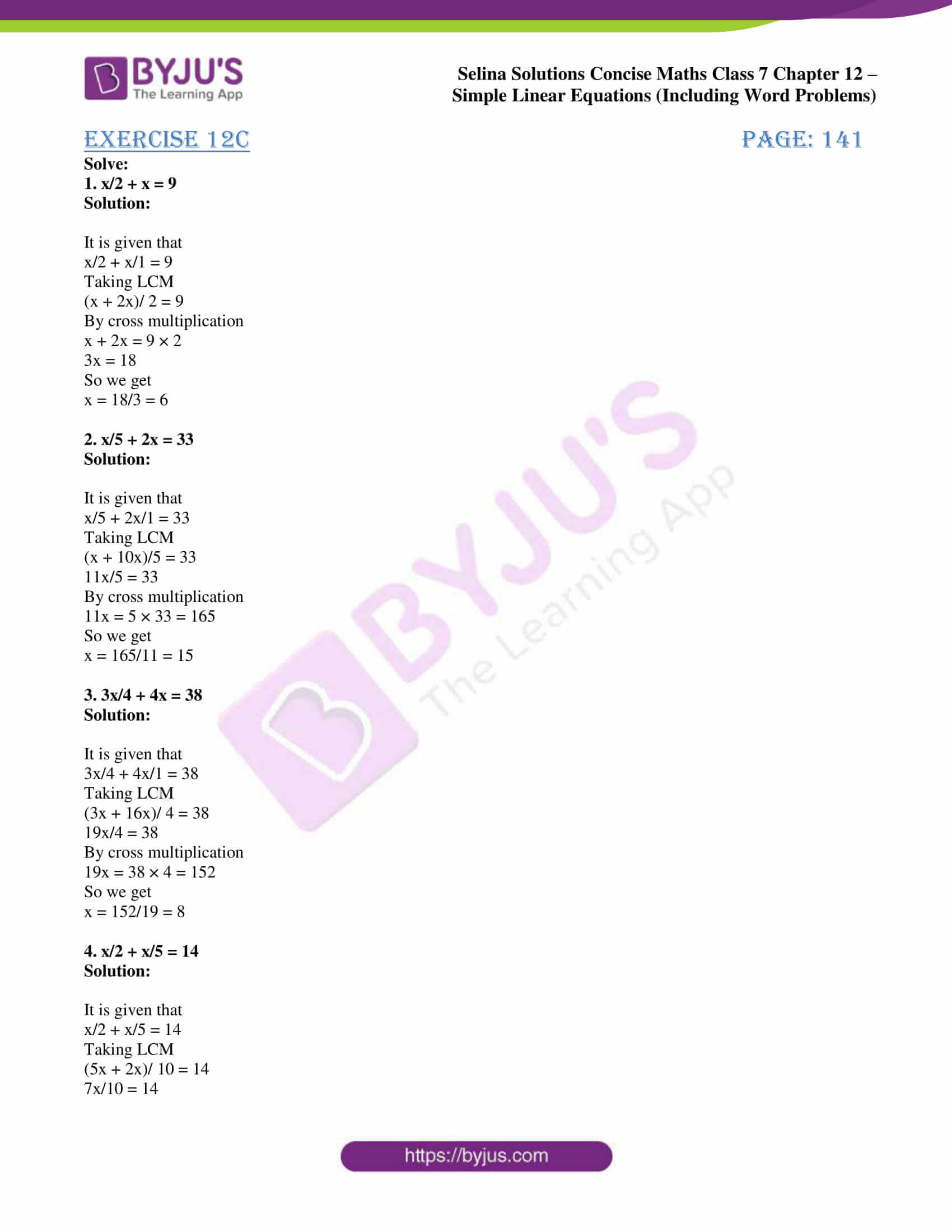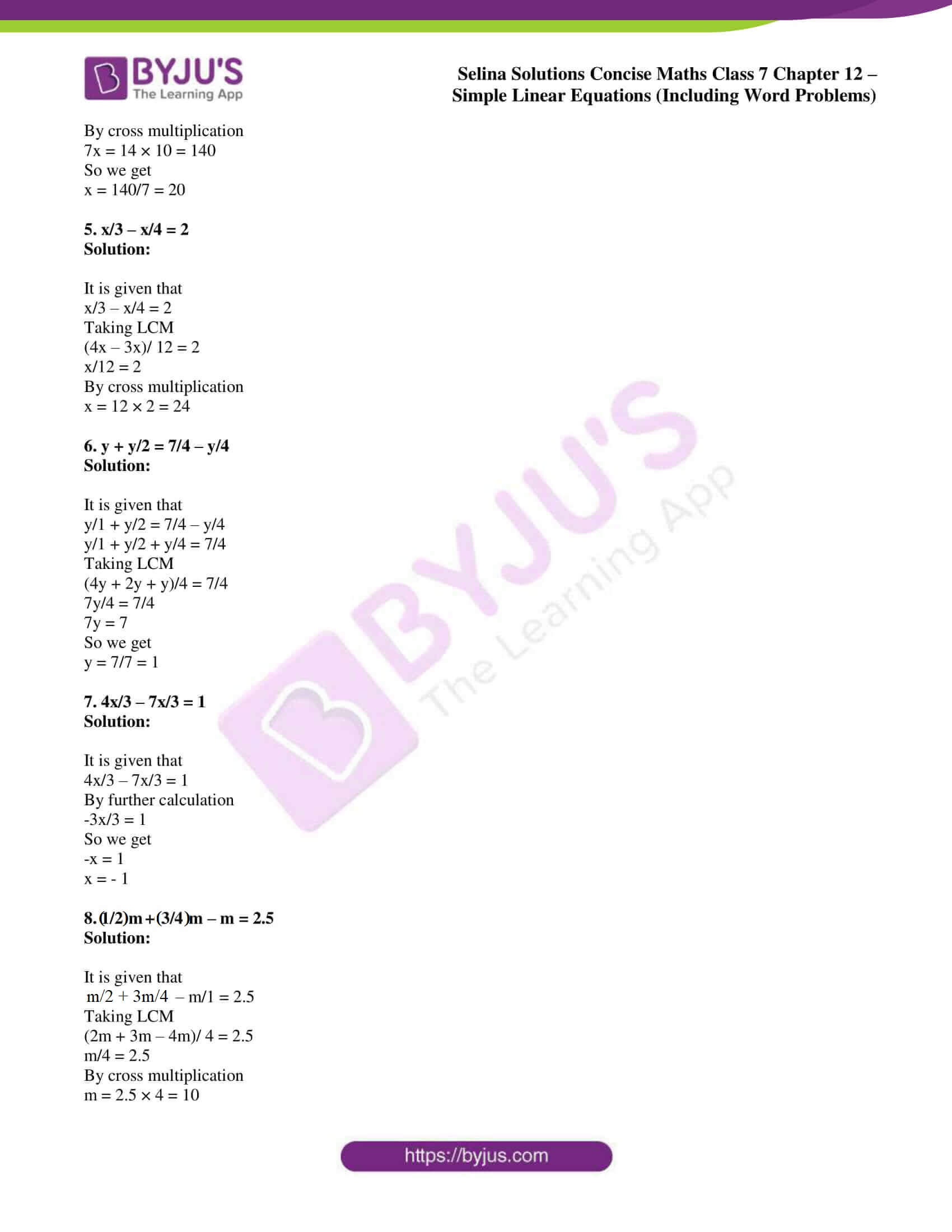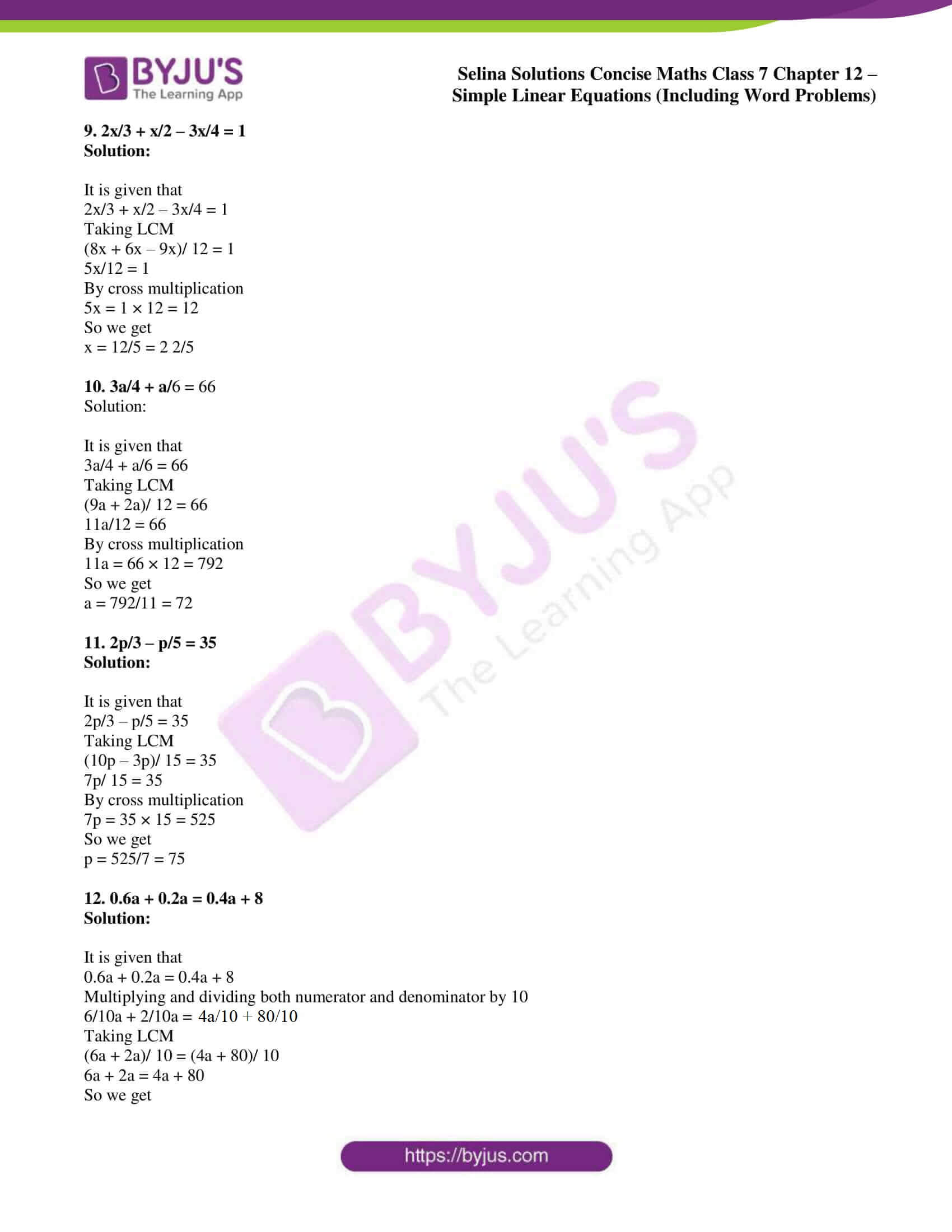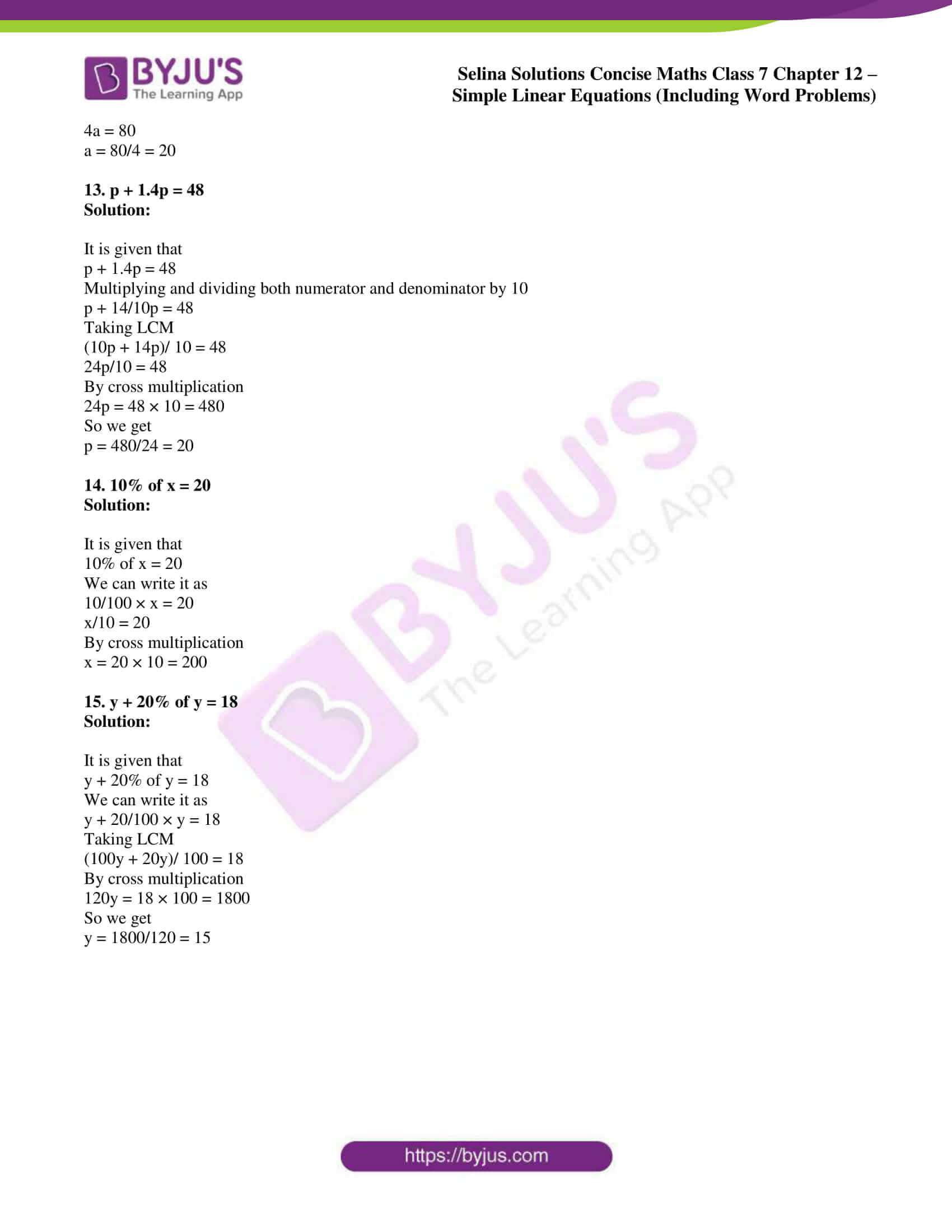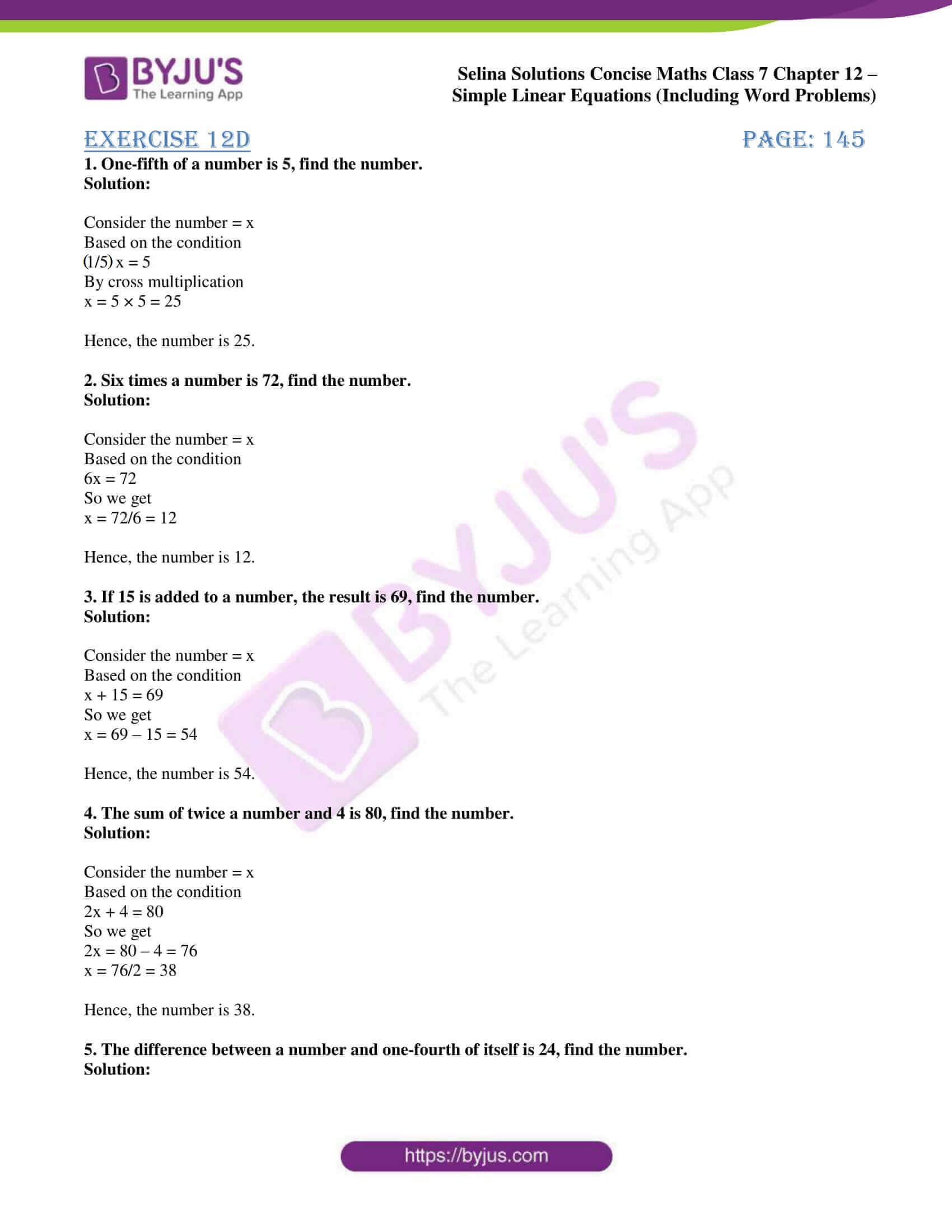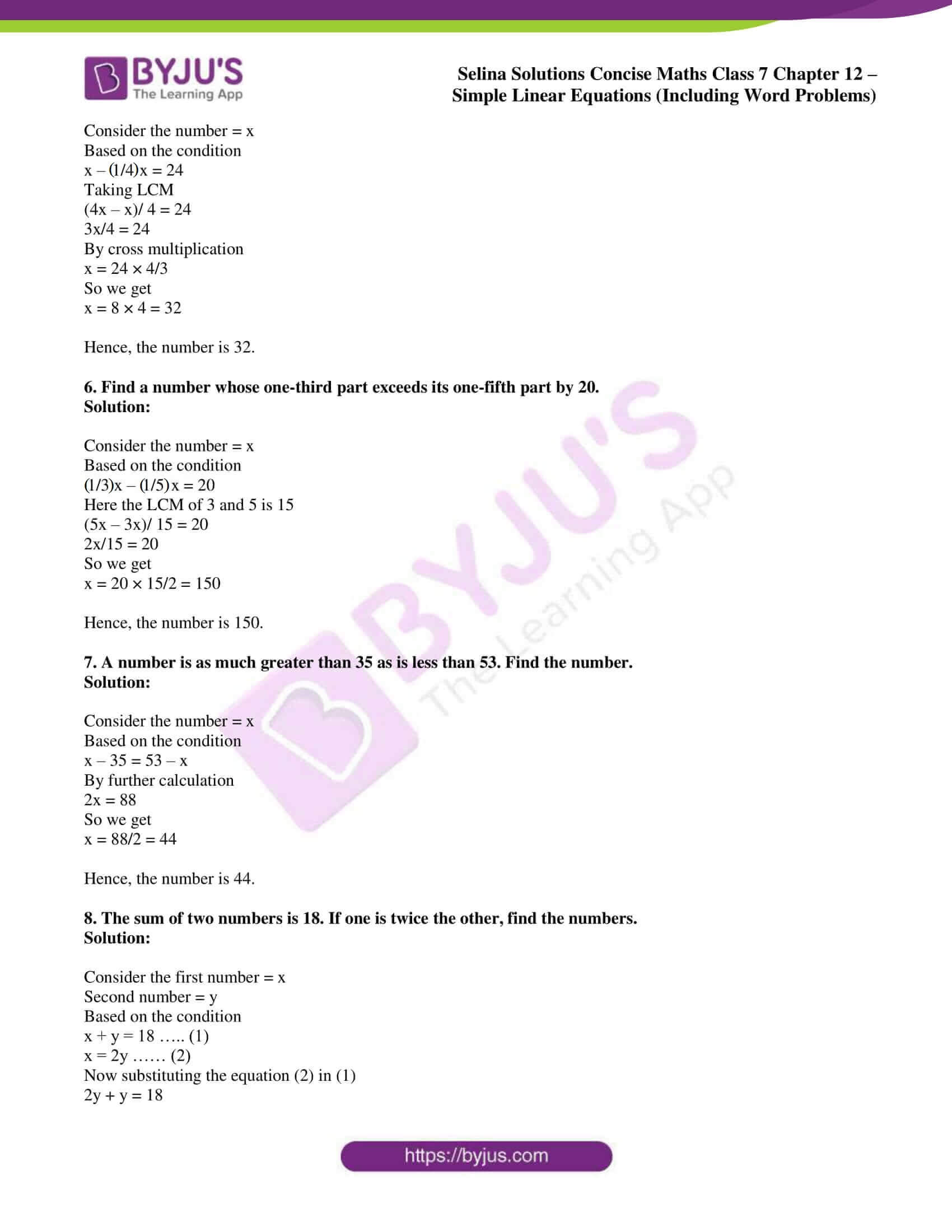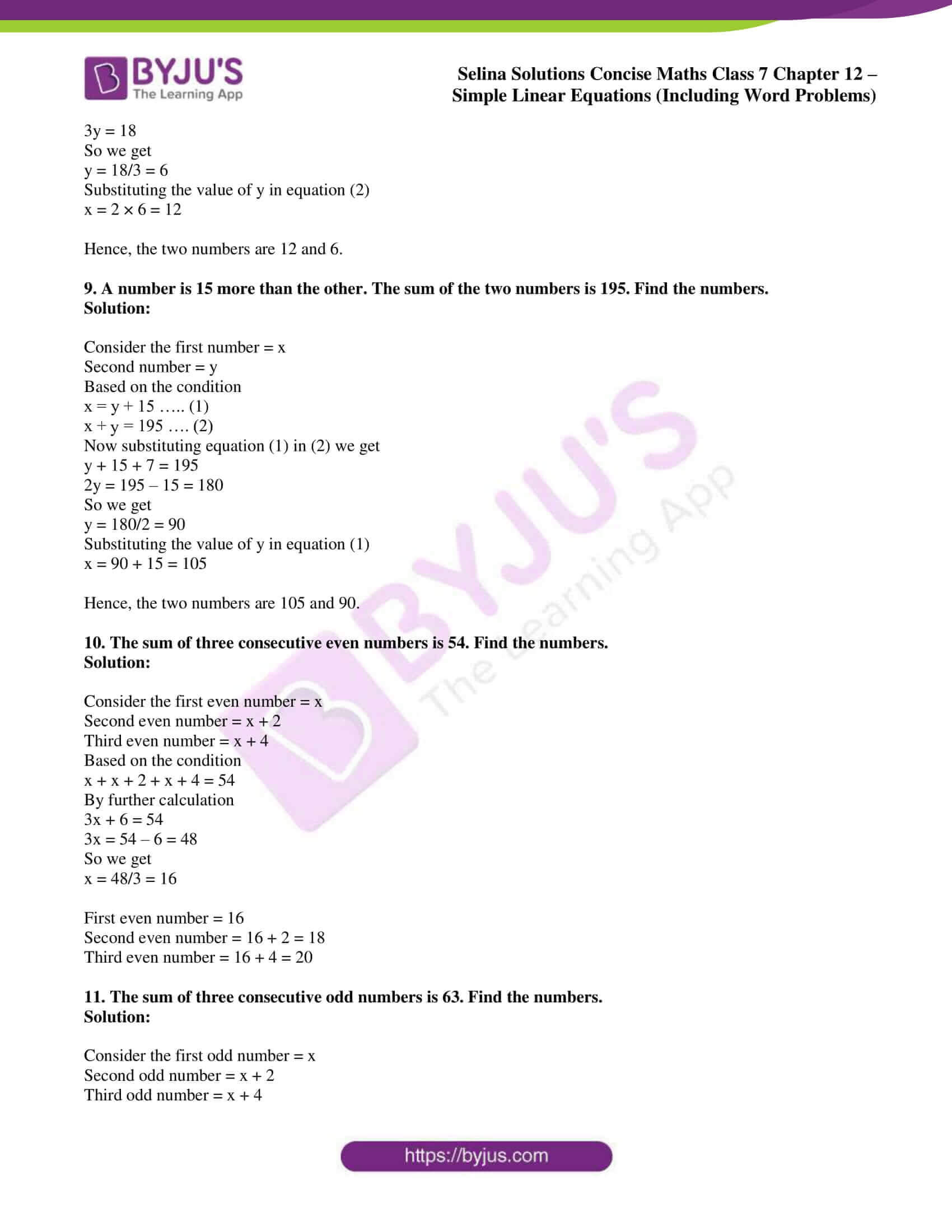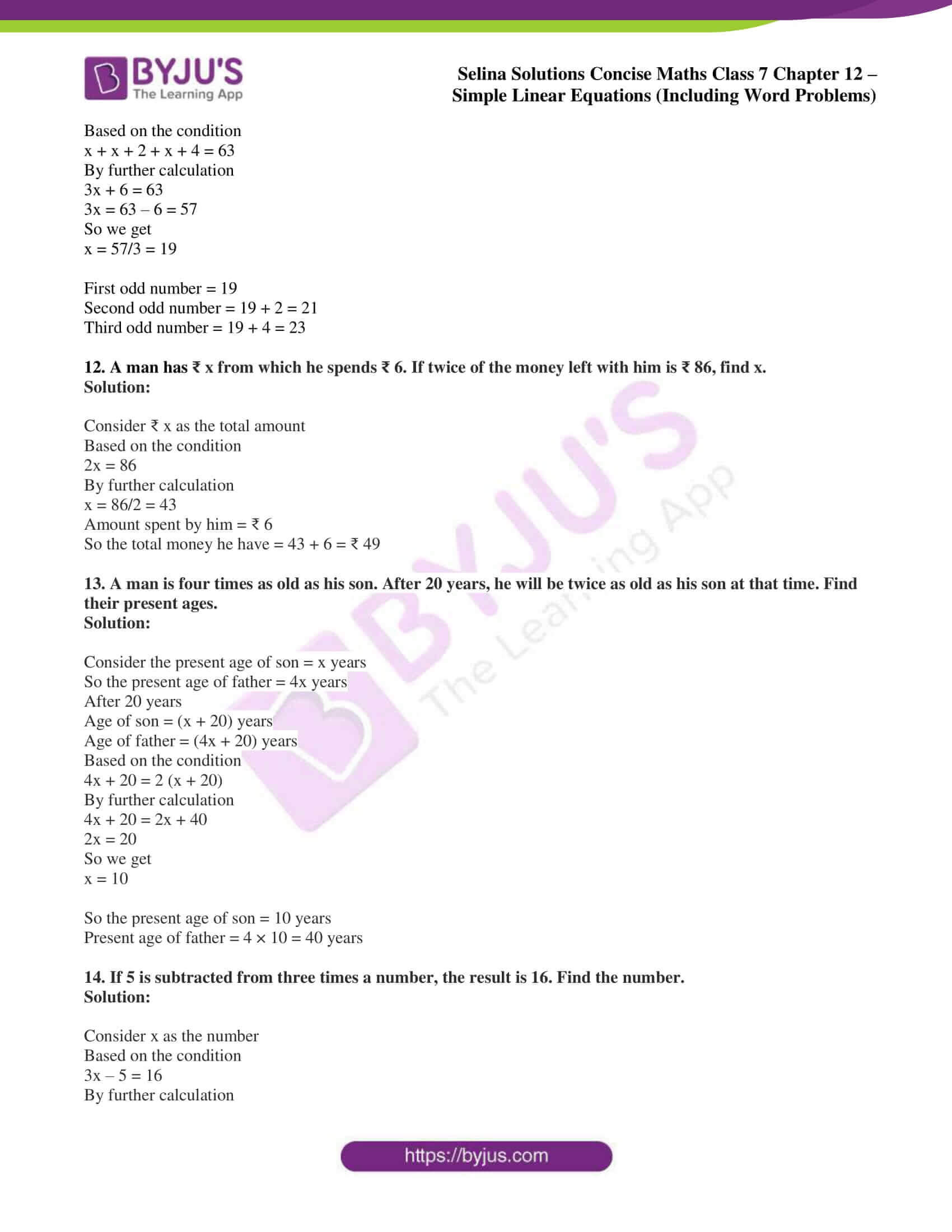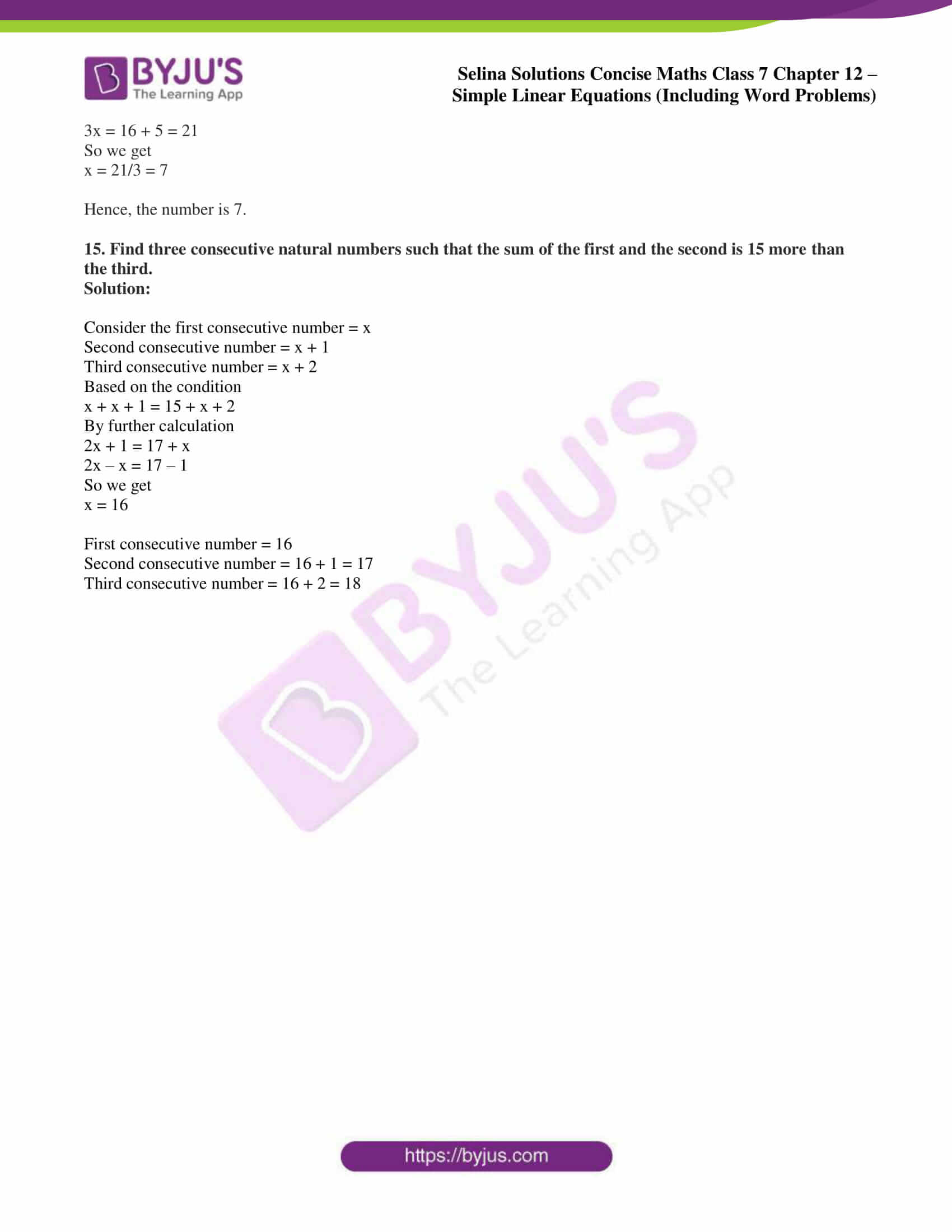### Exercises of Selina Solutions Concise Maths Class 7 Chapter 12 – Simple Linear Equations (Including Word Problems)

Exercise 12A Solutions

Exercise 12B Solutions

Exercise 12C Solutions

Exercise 12D Solutions

## Access Selina Solutions Concise Maths Class 7 Chapter 12: Simple Linear Equations (Including Word Problems)

#### Exercise 12A page: 139

Solve the following questions:

1. x + 5 = 10

Solution:

x + 5 = 10

So we get

x = 10 – 5 = 5

2. 2 + y = 7

Solution:

2 + y = 7

So we get

y = 7 – 2 = 5

3. a – 2 = 6

Solution:

a – 2 = 6

So we get

a = 6 + 2 = 8

4. x – 5 = 8

Solution:

x – 5 = 8

So we get

x = 8 + 5 = 13

5. 5 – d = 12

Solution:

5 – d = 12

So we get

– d = 12 – 5 = 7

d = -7

6. 3p = 12

Solution:

3p = 12

So we get

p = 12/3 = 4

7. 14 = 7m

Solution:

14 = 7m

So we get

m = 14/7 = 2

8. 2x = 0

Solution:

2x = 0

So we get

x = 0/2 = 0

9. x/9 = 2

Solution:

x/9 = 2

So we get

x = 2 × 9 = 18

10. y/-12 = -4

Solution:

y/-12 = -4

So we get

y = -4 × -12 = 48

11. 8x – 2 = 38

Solution:

8x – 2 = 38

So we get

8x = 38 + 2

8x = 40

x = 40/8 = 5

12. 2x + 5 = 5

Solution:

2x + 5 = 5

So we get

2x = 5 – 5

2x = 0

x = 0/2 = 0

13. 5x – 1 = 74

Solution:

5x – 1 = 74

So we get

5x = 74 + 1

5x = 75

x = 75/5 = 15

14. 14 = 27 – x

Solution:

14 = 27 – x

So we get

x = 27 – 14 = 13

15. 10 + 6a = 40

Solution:

10 + 6a = 40

So we get

6a = 40 – 10

6a = 30

a = 30/6 = 5

#### Exercise 12B page: 140

Solve:

1. 8y – 4y = 20

Solution:

8y – 4y = 20

By further calculation

4y = 20

So we get

y = 20/4 = 5

2. 9b – 4b + 3b = 16

Solution:

9b – 4b + 3b = 16

By further calculation

8b = 16

So we get

b = 16/8 = 2

3. 5y + 8 = 8y – 18

Solution:

5y + 8 = 8y – 18

By further calculation

8y – 5y = 8 + 18

So we get

3y = 26

y = 26/3 = 8 2/3

4. 6 = 7 + 2p – 5

Solution:

6 = 7 + 2p – 5

By further calculation

2p = 6 – 7 + 5

So we get

2p = 4

p = 4/2 = 2

5. 8 – 7x = 13x + 8

Solution:

8 – 7x = 13x + 8

By further calculation

13x + 7x = 8 – 8

20x = 0

x = 0/20 = 0

6. 4x – 5x + 2x = 28 + 3x

Solution:

4x – 5x + 2x = 28 + 3x

By further calculation

4x – 5x + 2x – 3x = 28

So we get

– 2x = 28

x = 28/-2 = – 14

7. 9 + m = 6m + 8 – m

Solution:

9 + m = 6m + 8 – m

By further calculation

6m – m – m = 9 – 8

4m = 1

So we get

m = 1/4

8. 24 = y + 2y + 3 + 4y

Solution:

24 = y + 2y + 3 + 4y

By further calculation

24 – 3 = 7y

21 = 7y

So we get

y = 21/7 = 3

9. 19x + 13 – 12x + 3 = 23

Solution:

19x + 13 – 12x + 3 = 23

By further calculation

7x = 23 – 16

So we get

7x = 7

x = 7/7 = 1

10. 6b + 40 = – 100 – b

Solution:

6b + 40 = – 100 – b

By further calculation

7b = – 100 – 40

7b = -140

So we get

b = -140/7 = – 20

11. 6 – 5m – 1 + 3m = 0

Solution:

6 – 5m – 1 + 3m = 0

By further calculation

5 – 2m = 0

So we get

2m = 5

m = 5/2 = 2 1/2

12. 0.4x – 1.2 = 0.3x + 0.6

Solution:

0.4x – 1.2 = 0.3x + 0.6

By further calculation

0.1x = 1.8

Multiply and divide both numerator and denominator by 10

1/10x = 18/10

By cross multiplication

x = 18/10 × 10/1 = 18

13. 6 (x + 4) = 36

Solution:

6 (x + 4) = 36

By further calculation

6x + 24 = 36

So we get

6x = 36 – 24

6x = 12

x = 12/6 = 2

14. 9 (a + 5) + 2 = 11

Solution:

9 (a + 5) + 2 = 11

By further calculation

9a + 45 + 2 = 11

So we get

9a = 11 – 47

9a = – 36

a = -36/9 = – 4

15. 4 (x – 2) = 12

Solution:

4 (x – 2) = 12

By further calculation

4x – 8 = 12

So we get

4x = 20

x = 20/4 = 5

#### Exercise 12C page: 141

Solve:

1. x/2 + x = 9

Solution:

It is given that

x/2 + x/1 = 9

Taking LCM

(x + 2x)/ 2 = 9

By cross multiplication

x + 2x = 9 × 2

3x = 18

So we get

x = 18/3 = 6

2. x/5 + 2x = 33

Solution:

It is given that

x/5 + 2x/1 = 33

Taking LCM

(x + 10x)/5 = 33

11x/5 = 33

By cross multiplication

11x = 5 × 33 = 165

So we get

x = 165/11 = 15

3. 3x/4 + 4x = 38

Solution:

It is given that

3x/4 + 4x/1 = 38

Taking LCM

(3x + 16x)/ 4 = 38

19x/4 = 38

By cross multiplication

19x = 38 × 4 = 152

So we get

x = 152/19 = 8

4. x/2 + x/5 = 14

Solution:

It is given that

x/2 + x/5 = 14

Taking LCM

(5x + 2x)/ 10 = 14

7x/10 = 14

By cross multiplication

7x = 14 × 10 = 140

So we get

x = 140/7 = 20

5. x/3 – x/4 = 2

Solution:

It is given that

x/3 – x/4 = 2

Taking LCM

(4x – 3x)/ 12 = 2

x/12 = 2

By cross multiplication

x = 12 × 2 = 24

6. y + y/2 = 7/4 – y/4

Solution:

It is given that

y/1 + y/2 = 7/4 – y/4

y/1 + y/2 + y/4 = 7/4

Taking LCM

(4y + 2y + y)/4 = 7/4

7y/4 = 7/4

7y = 7

So we get

y = 7/7 = 1

7. 4x/3 – 7x/3 = 1

Solution:

It is given that

4x/3 – 7x/3 = 1

By further calculation

-3x/3 = 1

So we get

-x = 1

x = – 1

8. (1/2)m + (3/4)m – m = 2.5

Solution:

It is given that

(1/2)m + (3/4)m – m/1 = 2.5

Taking LCM

(2m + 3m – 4m)/ 4 = 2.5

m/4 = 2.5

By cross multiplication

m = 2.5 × 4 = 10

9. 2x/3 + x/2 – 3x/4 = 1

Solution:

It is given that

2x/3 + x/2 – 3x/4 = 1

Taking LCM

(8x + 6x – 9x)/ 12 = 1

5x/12 = 1

By cross multiplication

5x = 1 × 12 = 12

So we get

x = 12/5 = 2 2/5

10. 3a/4 + a/6 = 66

Solution:

It is given that

3a/4 + a/6 = 66

Taking LCM

(9a + 2a)/ 12 = 66

11a/12 = 66

By cross multiplication

11a = 66 × 12 = 792

So we get

a = 792/11 = 72

11. 2p/3 – p/5 = 35

Solution:

It is given that

2p/3 – p/5 = 35

Taking LCM

(10p – 3p)/ 15 = 35

7p/ 15 = 35

By cross multiplication

7p = 35 × 15 = 525

So we get

p = 525/7 = 75

12. 0.6a + 0.2a = 0.4a + 8

Solution:

It is given that

0.6a + 0.2a = 0.4a + 8

Multiplying and dividing both numerator and denominator by 10

6/10a + 2/10a = 4a/10 + 80/10

Taking LCM

(6a + 2a)/ 10 = (4a + 80)/ 10

6a + 2a = 4a + 80

So we get

4a = 80

a = 80/4 = 20

13. p + 1.4p = 48

Solution:

It is given that

p + 1.4p = 48

Multiplying and dividing both numerator and denominator by 10

p + 14/10p = 48

Taking LCM

(10p + 14p)/ 10 = 48

24p/10 = 48

By cross multiplication

24p = 48 × 10 = 480

So we get

p = 480/24 = 20

14. 10% of x = 20

Solution:

It is given that

10% of x = 20

We can write it as

10/100 × x = 20

x/10 = 20

By cross multiplication

x = 20 × 10 = 200

15. y + 20% of y = 18

Solution:

It is given that

y + 20% of y = 18

We can write it as

y + 20/100 × y = 18

Taking LCM

(100y + 20y)/ 100 = 18

By cross multiplication

120y = 18 × 100 = 1800

So we get

y = 1800/120 = 15

#### Exercise 12D page: 145

1. One-fifth of a number is 5, find the number.

Solution:

Consider the number = x

Based on the condition

(1/5)x = 5

By cross multiplication

x = 5 × 5 = 25

Hence, the number is 25.

2. Six times a number is 72, find the number.

Solution:

Consider the number = x

Based on the condition

6x = 72

So we get

x = 72/6 = 12

Hence, the number is 12.

3. If 15 is added to a number, the result is 69, find the number.

Solution:

Consider the number = x

Based on the condition

x + 15 = 69

So we get

x = 69 – 15 = 54

Hence, the number is 54.

4. The sum of twice a number and 4 is 80, find the number.

Solution:

Consider the number = x

Based on the condition

2x + 4 = 80

So we get

2x = 80 – 4 = 76

x = 76/2 = 38

Hence, the number is 38.

5. The difference between a number and one-fourth of itself is 24, find the number.

Solution:

Consider the number = x

Based on the condition

x – (1/4)x = 24

Taking LCM

(4x – x)/ 4 = 24

3x/4 = 24

By cross multiplication

x = 24 × 4/3

So we get

x = 8 × 4 = 32

Hence, the number is 32.

6. Find a number whose one-third part exceeds its one-fifth part by 20.

Solution:

Consider the number = x

Based on the condition

(1/3)x – (1/5)x = 20

Here the LCM of 3 and 5 is 15

(5x – 3x)/ 15 = 20

2x/15 = 20

So we get

x = 20 × 15/2 = 150

Hence, the number is 150.

7. A number is as much greater than 35 as is less than 53. Find the number.

Solution:

Consider the number = x

Based on the condition

x – 35 = 53 – x

By further calculation

2x = 88

So we get

x = 88/2 = 44

Hence, the number is 44.

8. The sum of two numbers is 18. If one is twice the other, find the numbers.

Solution:

Consider the first number = x

Second number = y

Based on the condition

x + y = 18 ….. (1)

x = 2y …… (2)

Now substituting the equation (2) in (1)

2y + y = 18

3y = 18

So we get

y = 18/3 = 6

Substituting the value of y in equation (2)

x = 2 × 6 = 12

Hence, the two numbers are 12 and 6.

9. A number is 15 more than the other. The sum of the two numbers is 195. Find the numbers.

Solution:

Consider the first number = x

Second number = y

Based on the condition

x = y + 15 ….. (1)

x + y = 195 …. (2)

Now substituting equation (1) in (2) we get

y + 15 + 7 = 195

2y = 195 – 15 = 180

So we get

y = 180/2 = 90

Substituting the value of y in equation (1)

x = 90 + 15 = 105

Hence, the two numbers are 105 and 90.

10. The sum of three consecutive even numbers is 54. Find the numbers.

Solution:

Consider the first even number = x

Second even number = x + 2

Third even number = x + 4

Based on the condition

x + x + 2 + x + 4 = 54

By further calculation

3x + 6 = 54

3x = 54 – 6 = 48

So we get

x = 48/3 = 16

First even number = 16

Second even number = 16 + 2 = 18

Third even number = 16 + 4 = 20

11. The sum of three consecutive odd numbers is 63. Find the numbers.

Solution:

Consider the first odd number = x

Second odd number = x + 2

Third odd number = x + 4

Based on the condition

x + x + 2 + x + 4 = 63

By further calculation

3x + 6 = 63

3x = 63 – 6 = 57

So we get

x = 57/3 = 19

First odd number = 19

Second odd number = 19 + 2 = 21

Third odd number = 19 + 4 = 23

12. A man has ₹ x from which he spends ₹ 6. If twice of the money left with him is ₹ 86, find x.

Solution:

Consider ₹ x as the total amount

Based on the condition

2x = 86

By further calculation

x = 86/2 = 43

Amount spent by him = ₹ 6

So the total money he have = 43 + 6 = ₹ 49

13. A man is four times as old as his son. After 20 years, he will be twice as old as his son at that time. Find their present ages.

Solution:

Consider the present age of son = x years

So the present age of father = 4x years

After 20 years

Age of son = (x + 20) years

Age of father = (4x + 20) years

Based on the condition

4x + 20 = 2 (x + 20)

By further calculation

4x + 20 = 2x + 40

2x = 20

So we get

x = 10

So the present age of son = 10 years

Present age of father = 4 × 10 = 40 years

14. If 5 is subtracted from three times a number, the result is 16. Find the number.

Solution:

Consider x as the number

Based on the condition

3x – 5 = 16

By further calculation

3x = 16 + 5 = 21

So we get

x = 21/3 = 7

Hence, the number is 7.

15. Find three consecutive natural numbers such that the sum of the first and the second is 15 more than the third.

Solution:

Consider the first consecutive number = x

Second consecutive number = x + 1

Third consecutive number = x + 2

Based on the condition

x + x + 1 = 15 + x + 2

By further calculation

2x + 1 = 17 + x

2x – x = 17 – 1

So we get

x = 16

First consecutive number = 16

Second consecutive number = 16 + 1 = 17

Third consecutive number = 16 + 2 = 18